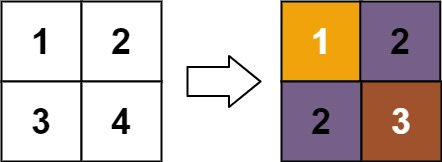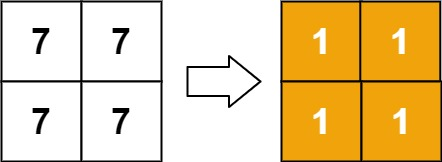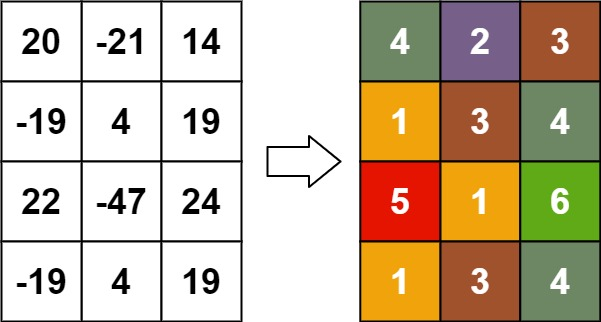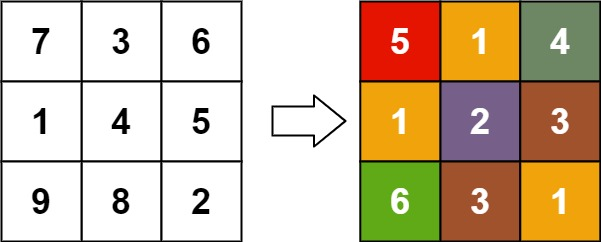# GeetCode Hub

Given an `m x n` `matrix`, return a new matrix `answer` where `answer[row][col]` is the rank of `matrix[row][col]`.

The rank is an integer that represents how large an element is compared to other elements. It is calculated using the following rules:

• The rank is an integer starting from `1`.
• If two elements `p` and `q` are in the same row or column, then:
• If `p < q` then `rank(p) < rank(q)`
• If `p == q` then `rank(p) == rank(q)`
• If `p > q` then `rank(p) > rank(q)`
• The rank should be as small as possible.

It is guaranteed that `answer` is unique under the given rules.

Example 1:```Input: matrix = [[1,2],[3,4]]
Output: [[1,2],[2,3]]
Explanation:
The rank of matrix is 1 because it is the smallest integer in its row and column.
The rank of matrix is 2 because matrix > matrix and matrix is rank 1.
The rank of matrix is 2 because matrix > matrix and matrix is rank 1.
The rank of matrix is 3 because matrix > matrix, matrix > matrix, and both matrix and matrix are rank 2.
```

Example 2:```Input: matrix = [[7,7],[7,7]]
Output: [[1,1],[1,1]]
```

Example 3:```Input: matrix = [[20,-21,14],[-19,4,19],[22,-47,24],[-19,4,19]]
Output: [[4,2,3],[1,3,4],[5,1,6],[1,3,4]]
```

Example 4:```Input: matrix = [[7,3,6],[1,4,5],[9,8,2]]
Output: [[5,1,4],[1,2,3],[6,3,1]]
```

Constraints:

• `m == matrix.length`
• `n == matrix[i].length`
• `1 <= m, n <= 500`
• `-109 <= matrix[row][col] <= 109`

class Solution { public int[][] matrixRankTransform(int[][] matrix) { } }